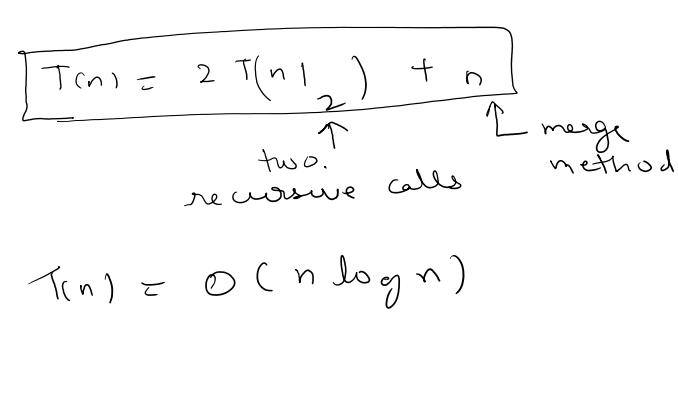life is too short for a diary

# Recursively sort a array

I recently came across a simple telephonic interview problem. The problem was to sort an array recursively. But even numbers need to be placed before odd numbers. At first glance it was obvious that it was a simple case of implementation of merge sort.

## Problem statement

Given an input array, produce the following output array

eg,

``````input array = [10 8 1 7 1 2]

output array = [2 8 10 1 1 7]
``````

## let’s use a simple template class

``````public class RecSortEven {
public static void main(String[] args) {
}
}
``````

## create a Array that needs to be sorted

``````public class RecSortEven {
public static void main(String[] args) {
List<Integer> testInput = new ArrayList<Integer>(Arrays.asList(10, 8, 1, 7, 1, 2));

/*sort the array recursively*/
cusSort(testInput);

}
}
``````

## Merge sort

If you are unfamiliary with merge sort, we just break the array into sub parts and later combine them based on our criteria. Here, we will maintiain left and right pointer which keeps track of the end of the array. Initially, the left is equal to 0 and right equals to the last element of the array.

``````/**
* calls merge sort
@param : testInput ArrayList
*/
public static void cusSort(List<Integer> testInput) {
mergeSort(testInput, 0, testInput.size() - 1);
}

``````

We calculate a mid point and recursively call the method twice one half of array and another half of array. lastly we add the crux of our logic in merge sort.

``````/* recursively sort the array
* @param testInput: ArrayList
* @param left integer
* @param right integer
*/
public static void mergeSort(List<Integer> testInput, int left, int right) {
//TODO: remember the base condition
if (left >= right) {
return;
}

int mid = left + (right - left) / 2;

mergeSort(testInput, left, mid );

mergeSort(testInput, mid + 1, right);

merge(testInput, left, mid, mid + 1, right);
}
``````

As per our requirement we need to sort even arrays before odd arrays, so we need to have a method to check that.

``````    /*
* checks if a number is even or not
* @param number integer
*/
public static boolean isEven(int number ) {
if (number % 2 == 0) {
return true;
} else {
return false;
}
}
``````

Lastly we need to write our merge method.

``````    /*combine arrays */
public static void merge(List<Integer> testInput, int left1, int right1, int left2, int right2) {

List<Integer> auxInput = new ArrayList<>();

int iter1 = left1, iter2 = left2;

while (iter1 <= right1 && iter2 <= right2) {

if (isEven(testInput.get(iter1)) && !isEven(testInput.get(iter2))) {
} else if (isEven(testInput.get(iter2)) && !isEven(testInput.get(iter1))) {
} else {

if (testInput.get(iter1) < testInput.get(iter2)) {
} else {
}
}
}

while (iter1 <= right1) {
}

while (iter2 <= right2) {
}

//copy the content
for (int i = left1; i <= right2; i++) {
testInput.set(i, auxInput.remove(0));
}

}

``````

## What is the complexity of the program

It’s a recursive algorithm , so we need a recurrence